# ShuffleNet: An Extremely Efficient Convolutional Neural Network for Mobile Devices

The ShuffleNet utilizes pointwise group convolution and channel shuffle to reduce computation cost while maintaining accuracy. It manages to obtain lower top-1 error than the MobileNet system on ImageNet classification, and achieves ~13x actual speedup over AlexNet while maintaining comparable accuracy.

Megvii Inc (a.k.a Face++) introduced ShuffleNet, which they claim to be an extremely computation efficient CNN architecture, designed for mobile devices with computing power of 10-150 MFLOPs. The ShuffleNet utilizes pointwise group convolution and channel shuffle to reduce computation cost while maintaining accuracy. It manages to obtain lower top-1 error than the MobileNet system on ImageNet classification, and achieves ~13x actual speedup over AlexNet while maintaining comparable accuracy.

### 1. Introduction

With the current trend of building deeper and larger CNNs, the most accurate CNNs usually consume billions of FLOPs. This paper, however, focuses on achieving the best accuracy with very limited computational budgets of about tens to hundreds of MFLOPs, targeting applications in drones, robots, phones, etc.

Since Xception and ResNeXt become less efficient in extremely small networks, the pointwise group convolution is proposed to reduce computation complexity. The main constituent architecture of ShuffleNet involves a novel channel shuffle operation used to help information flow across feature channels. The paper also presents the model evaluation on the ImageNet classification and MS COCO object detection tasks, and the speedup examination on real hardware.

### 2. Configurations

2.1 Channel Shuffle for Group Convolutions
Xception and ResNeXt balance an excellent trade-off between representation capability and computational cost, by introducing depthwise separable convolutions or group convolutions into the building blocks, but cannot fully take the 1×1 convolutions, called pointwise convolutions, into account. In tiny networks, expensive pointwise convolutions result in limited number of channels to meet the complexity constraint, which might significantly damage accuracy.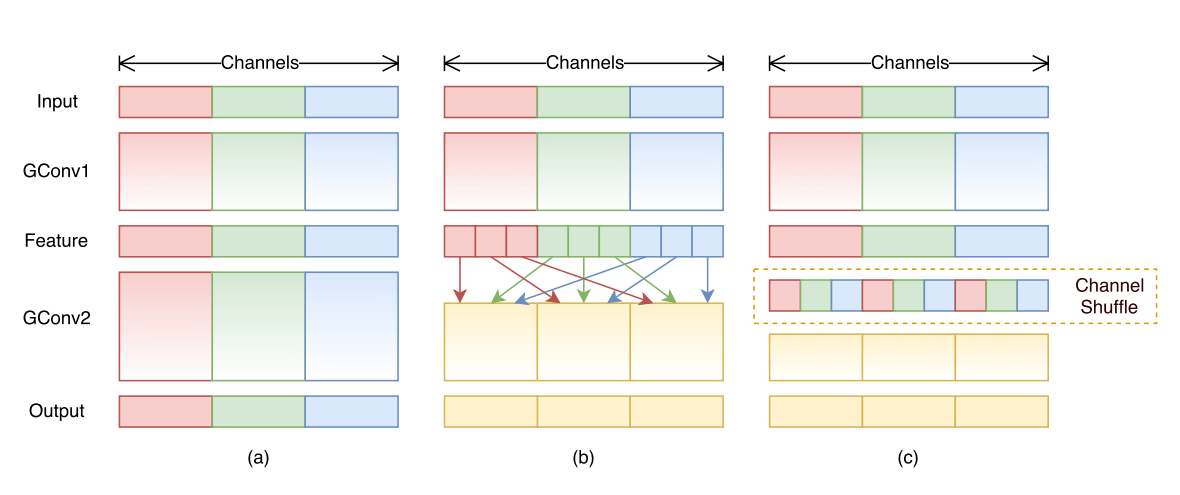Figure 1

In this section, the group convolutions are given as examples to demonstrate its capability in reducing computation cost.

Figure 1(a) illustrates a situation of two stacked group convolution layers, which has twofold properties, blocking the information flow between channel groups and weakening representations.

Figure 1(b) shows that the group convolution is allowed to obtain input data from different groups. Notice that the input channels are fully related to the output ones.

Figure 1(c) sets up the feature map from the previous group layer, which is then implemented by a channel shuffle operation. Channel shuffle operation is able to construct more powerful structures with multiple group convolutional layers.

The configuration in Figure 1(c) is preferable, because the channel shuffle operation still applies in the stacked layers, though the convolutions in Figure 1(b) have different number of groups. Additionally, it is differentiable so it is embedded into network structures.

2.2 ShuffleNet Unit
In this section, the ShuffleNet unit is presented. It is specially designed for small networks.Figure 2

The figurative comparisons of among those three configurations are obvious in Figure 2.
Figure 2(a) is a bottleneck unit with depthwise convolution (3×3 DWConv).
Figure 2(b) is a ShuffleNet unit with pointwise group convolution and channel shuffle.

The purpose of the second pointwise group convolution is to recover the channel dimension to match the shortcut path.

Figure 2(c) is a ShuffleNet unit with stride of 2.
Because of the pointwise group convolution with channel shuffle, all components in ShuffleNet unit can be computed efficiently.

2.3 Network Architecture

The overall ShuffleNet architecture is presented in Table 1. It is composed of a stack of ShuffleNet units grouped into three stages. In Table 1, the group number g controls the connection sparsity of pointwise convolutions. At the same time, g is assigned to be different numbers, so the output channels can be computed and evaluated to ensure the total computational costs are approximately the same(~140 MFLOPs).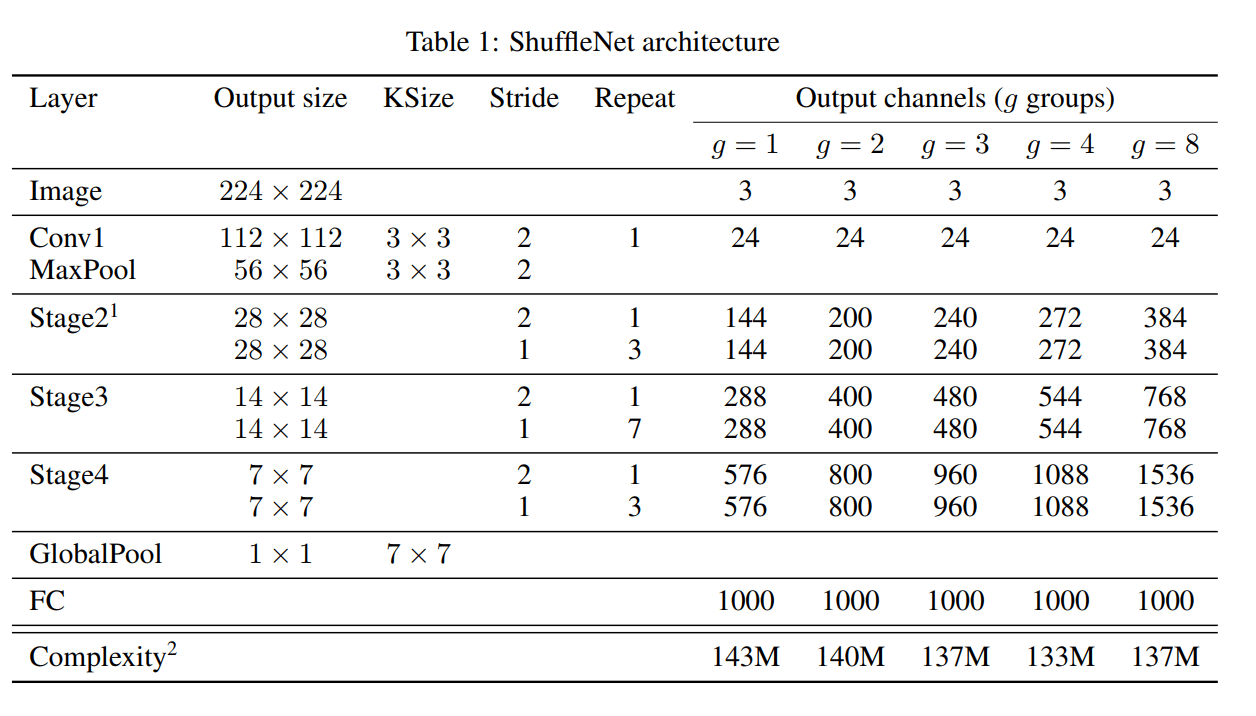The network can be freely customized to a desired complexity. To accomplish this, simply apply a scale factor s on the number of channels. For example, if the networks are denoted in Table 1 as “ShuffleNet 1×”, then “ShuffleNet s×” means scaling the number of filters in ShuffleNet 1× by s times, thus overall complexity will be roughly s squared times of ShuffleNet 1×.

### 3. Experiments

The proposed model is evaluated on the ImageNet 2016 classification dataset.

3.1 Ablation Study

The main concept of ShuffleNet is to apply pointwise group convolution and channel shuffle operation in the proposed network architecture, which are evaluated respectively.

3.1.1 On the Importance of Pointwise Group Convolutions

Table 2 shows the comparison results of ShuffleNet models of the same complexity, whose numbers of groups range from 1 to 8. If the group number equals 1, no pointwise group convolution is involved, and thus the ShuffleNet unit becomes an “Xception-like”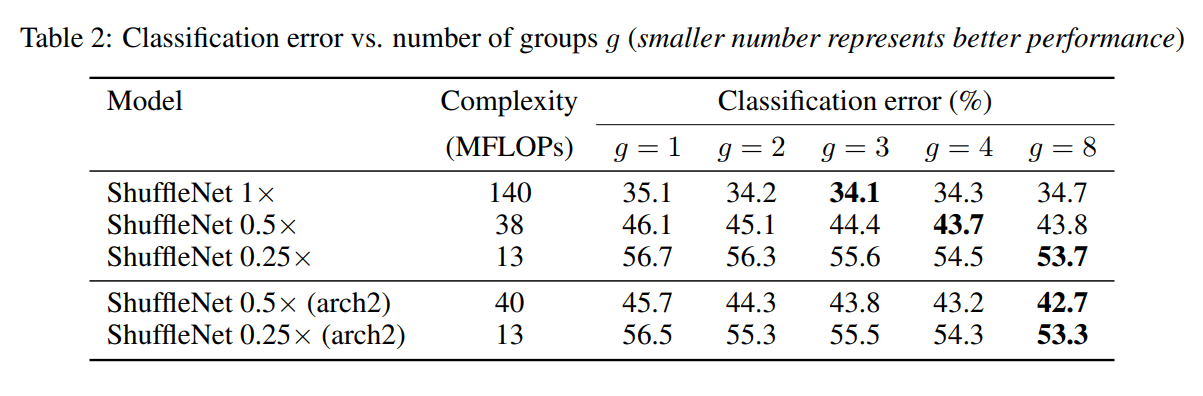From the result in Table 2, notice that the models with group convolutions (g > 1) have the better performance than those without pointwise group convolutions (g = 1), which indicates that smaller models are preferred. In this case, a smaller network involves thinner feature maps, so it benefits more from enlarged feature maps.

Table 2 also shows that the classification score may drop for a large group number g (e.g. g=4 or 8). Increasing group number g causes the decreasing number of the input channels for each convolutional filter, which may harm representation capability.

3.1.2 Channel Shuffle vs. No Shuffle

The shuffle operation proposed in this paper is to enable cross-group information flow for multiple group convolution layers, summarized in Table 3.
Table 3 demonstrates the performances of different ShuffleNet structures with and without channel shuffle.The result in Table 3 clearly shows a trend, where the classification scores increases with the models of a greater group number g. In addition, the ShuffleNet with channel shuffle has lower classification error, which proves the importance of cross-group information interchange.

3.2 Comparison with other Structure Units

Table 4 summarizes the results of the same training settings to train different models.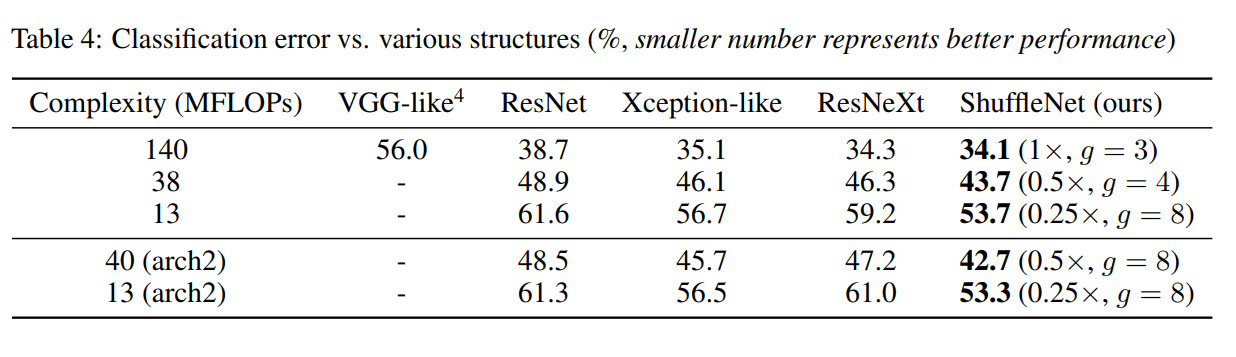ShuffleNet models outperform most others by a significant margin under different complexities.

3.3 Comparison with MobileNets and Other Frameworks

Table 5 demonstrates the classification scores under a variety of complexity levels.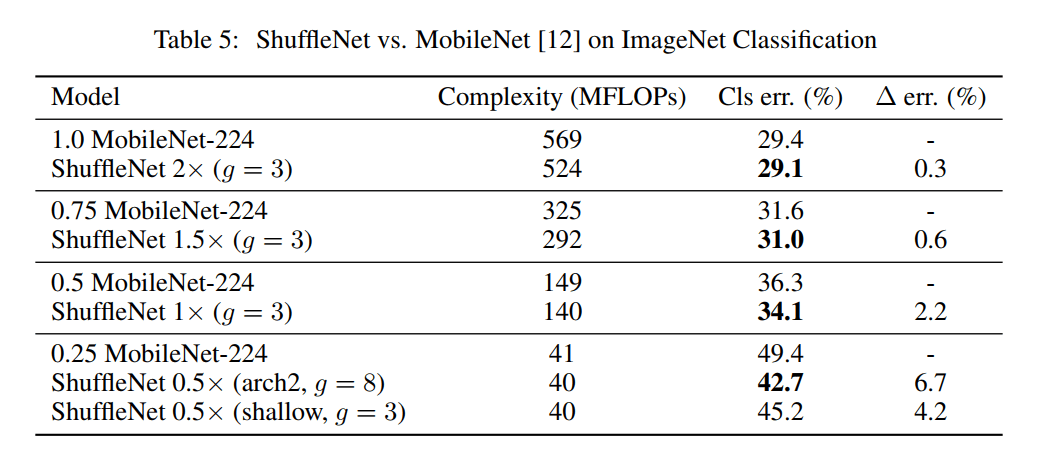The result demonstrates that ShuffleNet models outperform MobileNet for all the complexities. The ShuffleNet network is, admittedly, designed for small models (< 150 MFLOPs), but it is still better than MobileNet if considering the computation cost. For smaller networks (~40 MFLOPs), ShuffleNet outperforms MobileNet by 6.7%. From a configuration perspective, ShuffleNet architecture contains 50 layers (or 44 layers for arch2), whereas MobileNet only has 28 layers.Table 6 shows that the ShuffleNet is much more efficient with similar accuracy.

3.4 Generalization Ability

In this section, the shuffleNet model is examined on the task of MS COCO object detection. Table 7 shows that ShuffleNet 2× is superior over MobileNet by a significant margin on both resolutions; ShuffleNet 1× also has comparable results with MobileNet on 600× resolution, but ~4× complexity reduction.3.5 Actual Speedup Evaluation

The final evaluation is to test the actual inference speed of ShuffleNet models on a mobile device with an ARM platform. Table 8 shows the resulting inference time on the mobile device. The result proves that ShuffleNet is much faster than previous AlexNet-level models or speedup approaches.Implementing neural networks on mobile devices will become the next stage in the field of AI, and ShuffleNet by Face++ is very well presented in this paper. With comparable computation cost and better performance, ShuffleNet will gain popularity in the CNN field for mobile devices. The experiments on pointwise group convolution and channel shuffle operation, in which ShuffleNet 1x (g =3) performs the best, and comparisons with other structure units, in which ShuffleNet outperforms 5% over other units on average, demonstrate that ShuffleNet is extremely superior to other existing models.

The comparison of classification error on actual mobile device between ShuffleNet and AlexNet is not apparently distinguishable. However, the computation cost has been tremendously reduced from AlexNet of 720 MFLOPs, to ShuffleNet 1x of 140 MFLOPs and 0.5x of 40 MFLOPs. Future work may choose to focus on optimization, and performance tests on different mobile devices. Apple and Google put in a lot of effort on their mobile platforms, so it might be an interesting topic to test performance or computation cost with different brands.

Author: Bin Liu | Editor: Haojin Yang